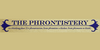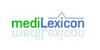VoltameterA voltameter or coulometer is a scientific instrument used for measuring quantity of electricity (electric charge). The SI unit of quantity of electricity is the coulomb. The voltameter should not be confused with a voltmeter, which measures electric potential. The SI unit for electric potential is the volt. ==Types of voltameter== The voltameter ...
Found on http://en.wikipedia.org/wiki/Voltameter

Voltameter• (n.) An instrument for measuring the voltaic electricity passing through it, by its effect in decomposing water or some other chemical compound acting as an electrolyte.
Found on http://thinkexist.com/dictionary/meaning/voltameter/

VoltameterVol·tam'e·ter noun [ Volta ic + - meter .] (Physics) An instrument for measuring the voltaic electricity passing through it, by its effect in decomposing water or some other chemical compound acting as an electrolyte.
Found on http://www.encyclo.co.uk/webster/V/35

voltameterinstrument for measuring electrical current indirectly
Found on http://phrontistery.info/v.html

voltameterType: Term Pronunciation: vōl-tam′ĕ-tĕr Definitions: 1. An apparatus for measuring the strength of a galvanic current by its electrolytic action.
Found on http://www.medilexicon.com/medicaldictionary.php?t=99365
No exact match found